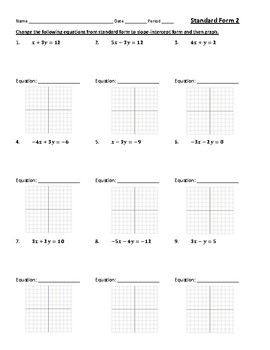# Standard Form of Linear Equations - Graphing, Converting to Slope InterceptSubject
Resource Type
Product Rating
File Type

PDF (Acrobat) Document File

Be sure that you have an application to open this file type before downloading and/or purchasing.

1 MB|4 pages
Share
Product Description

This is actually 2 days worth of worksheets that I used with my Algebra 1 students to teach Standard Form of Linear Equations. Depending on the level of students, this may be condensed into 1 day, although for most of my students two days was ideal.

On Standard Form 1, the worksheet starts with the x/y intercepts on graphs. Students use these to write the equation of the lines in slope/intercept form. Having students know what the x/y intercepts are is vital to understanding linear equations. The students then are given the x/y intercepts, and they have to graph them themselves and then write the equation. Finally, students are given an equation written in standard form, and students have to find the intercepts and then graph the line. This progression through intercepts made a lot of sense for my students.

On Standard Form 2, students are given problems that can't be solved by using the intercept method. I always teach my students to check for intercepts first to see if whole number intercepts are easily obtained. If not, they need to convert the equation to slope-intercept form and then graph. On this worksheet, students need to convert standard form to slope-intercept and then graph.

My students had a great understanding of graphing linear equations from standard form by both methods after these two worksheets. I provided answer keys to these worksheets.

Total Pages
4 pages